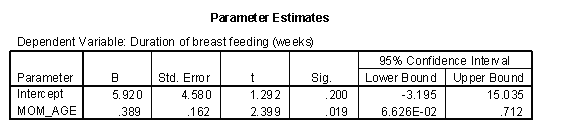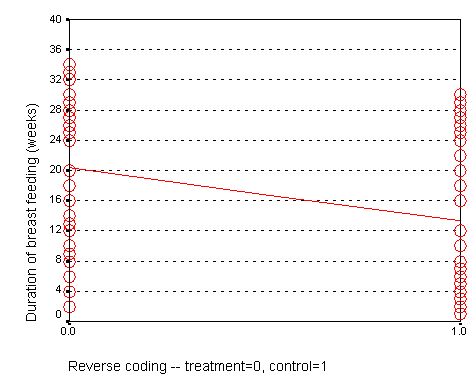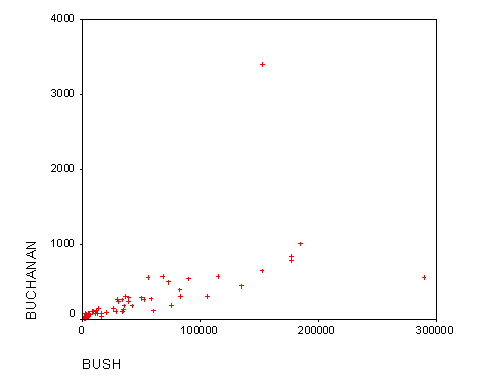# Interpreting coefficients in a linear regression model

## 2002-06-24

When I ask most people to remember their high school algebra class, I get a mixture of reactions. Most recoil in horror. About one in every four people say they liked that class. Personally, I thought that algebra, and all the other math classes I took were great because they didn’t require writing a term paper.

### Algebra formula for a straight line

One formula in algebra that most people can recall is the formula for a straight line. Actually, there are several different formulas, but the one that most people cite is

Y = m X + b

where m represents the slope, and b represents the y-intercept (we’ll call it just the intercept here). They can also sometimes remember the formula for the slope:

$m = \increment y / \increment x$

In English, we would say that this is the change in y divided by the change in x.

### Linear regression interpretation of a straight line

In linear regression, we use a straight linear to estimate a trend in data. We can’t always draw a straight line that passes through every data point, but we can find a line that “comes close” to most of the data. This line is an estimate, and we interpret the slope and the intercept of this line as follows:

The slope represents the estimated average change in Y when X increases by one unit. | The intercept represents the estimated average value of Y when X equals zero. |

Be cautious with your interpretation of the intercept. Sometimes the value X=0 is impossible, implausible, or represents a dangerousextrapolation outside the range of the data.

### First regression example with interpretation

The graph shown below represents the relationship between mother’s age and the duration of breast feeding in a research study on breast feeding in pre-term infants.The regression coefficients are shown below. The intercept, 6, is represented the estimated average duration of breast feeding for a mother that is zero years old. This is an impossible value, so the interpretation is not useful. What is useful, is the interpretation of the slope, approximately 0.4. The estimated average duration of breast feeding increases by 0.4 weeks for every extra year in the mother’s age.### Interpretation when X is categorical

When X is categorical, the interpretation changes somewhat. Let’s look at the simplest situation, a binary variable. A binary variable can have only two possible categories. Some examples are live/dead, treatment/control, diseased/healthy, male/female. We need to assign number codes to the categories. Most people assign the codes 1 and 2, but it is actually better to assign the codes 0 and 1.

When we represent a binary variable using 0-1 coding, the slope represents the estimated average change in Y when you switch from one group to the other. The intercept represents the estimated average value of Y for the group coded as zero.

The interpretation of the regression coefficient for a categorical variable with more than two values is a bit trickier, and we will discuss it in a separate handout.

### Second regression example with interpretation

In a study of breast feeding, we have a treatment group and a control group. Let us label the treatment group as 1 and the control group as 0. The outcome variable is the age when breast feeding stopped.The control group had a mean duration of breast feeding just a bit larger than 13. The mean for the treatment group is just a bit larger than 20. Notice that the regression line shown above connects the two means.In this situation, the intercept, 13, represents the average duration for the control group. The slope is 7, which is the change in the average duration when we move from the control group to the treatment group.

We could have just as easily labeled the treatment group as 0 and the control group as 1. If we did that, we would get a graph that looks like the following:Here, the intercept, 20, represents the mean of the treatment group. The slope, -7, represents the change in average duration as we move from the treatment group to the control group. It is actually this reverse coding that SPSS chooses as a default.Neither coding is correct or incorrect. Just make sure that you understand the difference. If you get a slope that is in the opposite direction of what you expected, perhaps it is because your software is using a different coding than what you expected.

### Final example: Florida election results

There has been much discussion of the unusual ballot format in Palm Beach County, Florida and how it may have led several thousand voters to cast their ballots for Buchanan rather than Gore. Greg Adams and Chris Fastnow (http://madison.hss.cmu.edu/) have performed a regression analysis of the county by county returns in Florida. A portion of their analyses is recomputed below using SPSS. My model is slightly different than the model of Adams and Fastnow, but it reaches the same general conclusion: the vote for Buchanan in Palm Beach County, Florida is a couple of thousand votes higher than you would expect, if it behaved similar to other Florida Counties.

The story behind the Palm Beach County vote is quite controversial. I discuss it, not to revive the controversy, but to illustrate some concepts in linear regression. Some background information is at:

The ballot in Palm Beach county was (according to some people) confusing, because it used a staggered two page format. The layout would lead some people who thought they were voting for Al Gore to instead cast a ballot for Patrick Buchanan instead. Exactly how confusing the ballot was and is still open to debate. Several statisticians offered analyses that tried to estimate how many votes for Buchanan might actually be votes for Gore. These analyses are far more detailed than what I present here. My analysis is more useful for helping you to understand concepts in regression than for resolving this voting controversy.The scatter plot shown above shows the number of votes for George Bush in each Florida County versus the number of votes for Patrick Buchanan in the same county.

The regression equation is:

Notice that 0.0049 is roughly 1/500. How do we interpret these numbers? The intercept is 45, which means that the estimated average number of votes for Buchanan would be 45 in a county with zero votes for Bush. This is an extrapolation, as every single county in Florida had thousands of votes for Bush. The slope is 1/200, which means that the estimated average number of votes for Buchanan increases by 1/200 for each additional vote for Bush. In other words, each additional 200 votes for Bush is associated with an increase of 1 vote for Buchanan.

We can compute a predicted number of votes for Buchanan for each county by using the above equation. Palm Beach County had 152,846 votes for Bush. So the regression model would predict that Buchanan should get:

45 + 0.0049 * 152,846 = 797.

Thus, if the relationship observed across the entire state held exactly in Palm Beach County, then we would estimate the vote count for Buchanan to be 797.

There were actually 3,407 votes recorded for Buchanan, which is quite a discrepancy from what we predicted. The residual, the difference between what we observed and what would be predicted by the regression model is:

3,407 - 797 = 2,610.

One possible interpretation is that this discrepancy represents an estimate of the number of people who voted incorrectly for Buchanan. Such an interpretation would have to consider other possibilities, though. Is there something unique about Palm Beach County that would cause that county to vote in disproportionate numbers for Buchanan? Buchanan does indeed have some relatives in the area, and although they do not number in the thousands, perhaps they exerted some influence on their community.

Other information might tend to corroborate that a large number of votes were cast erroneously for Buchanan. Some of the highest vote counts for Buchanan were in precincts that were most heavily Democratic. There were also a large number of complaints received by the election board prior to anyone knowing how close the vote count in Florida would be.

There are other models that have been considered for the Palm Beach County vote, and most of them show a similar size discrepancy between the observed vote and the vote that would be predicted the regression model. It would set a dangerous precedent, of course, to use a statistical model to adjust vote counts, so this example is more for understanding what might have gone wrong and the magnitude of the error made.

You can find an earlier version of this page on my original website.